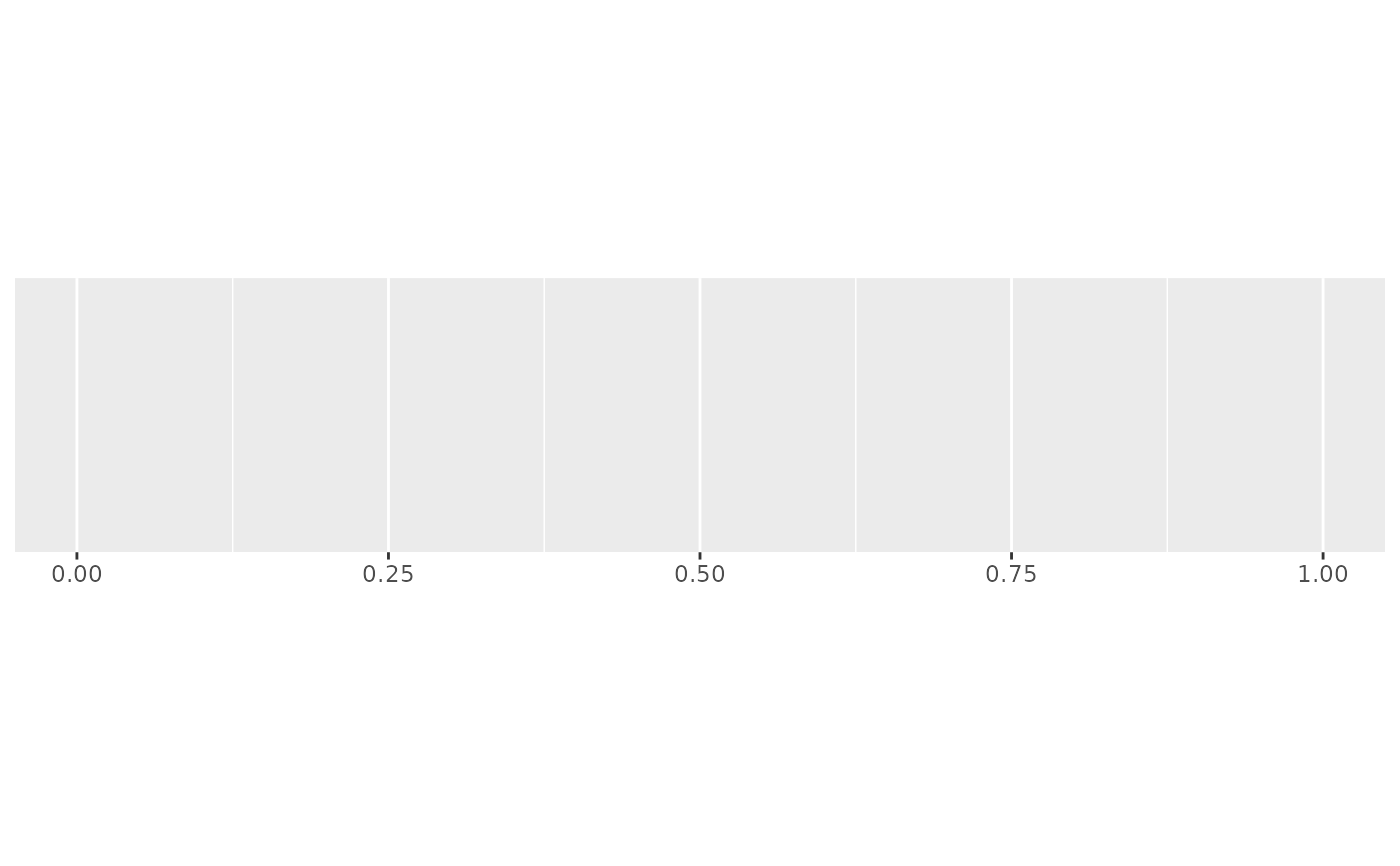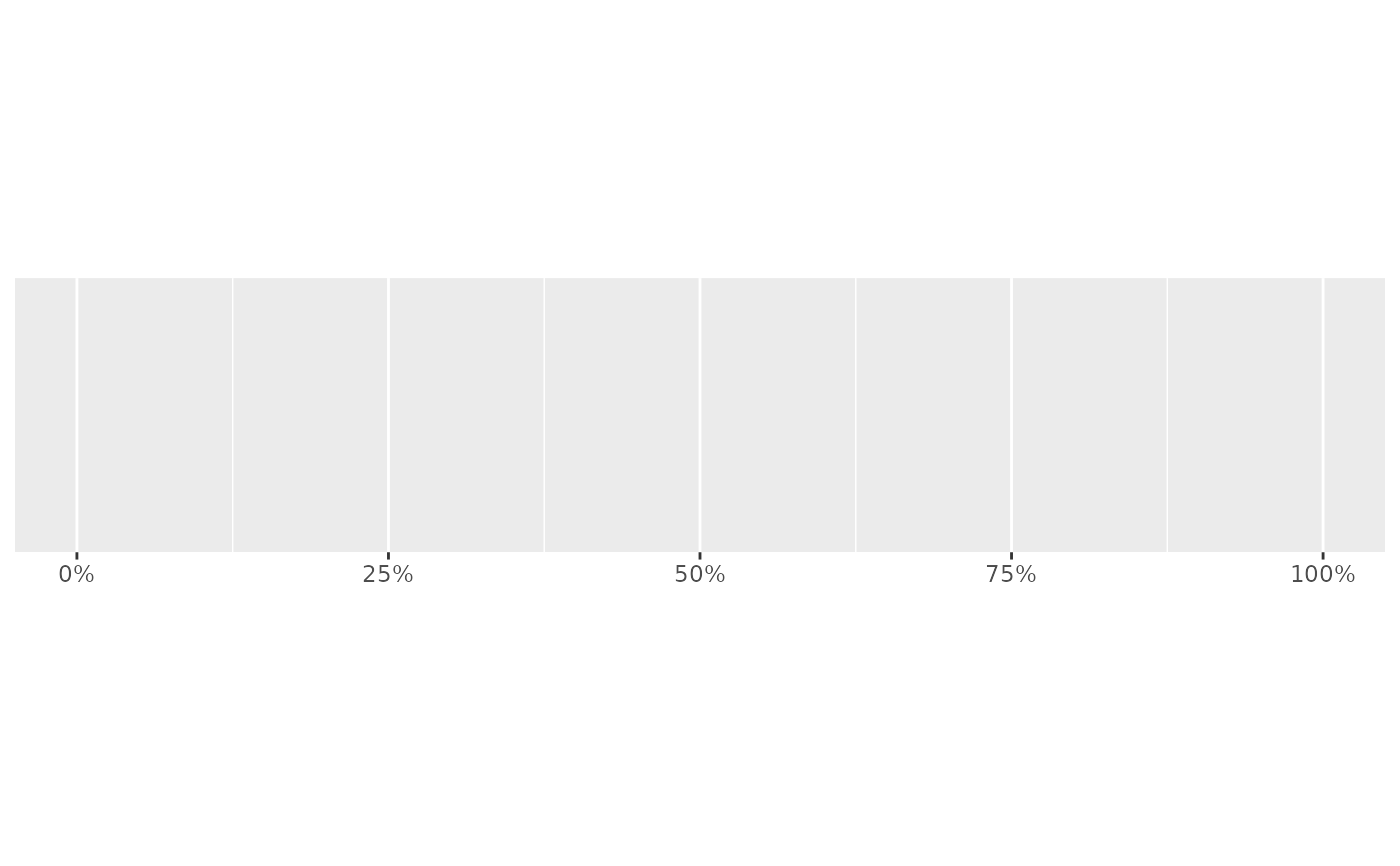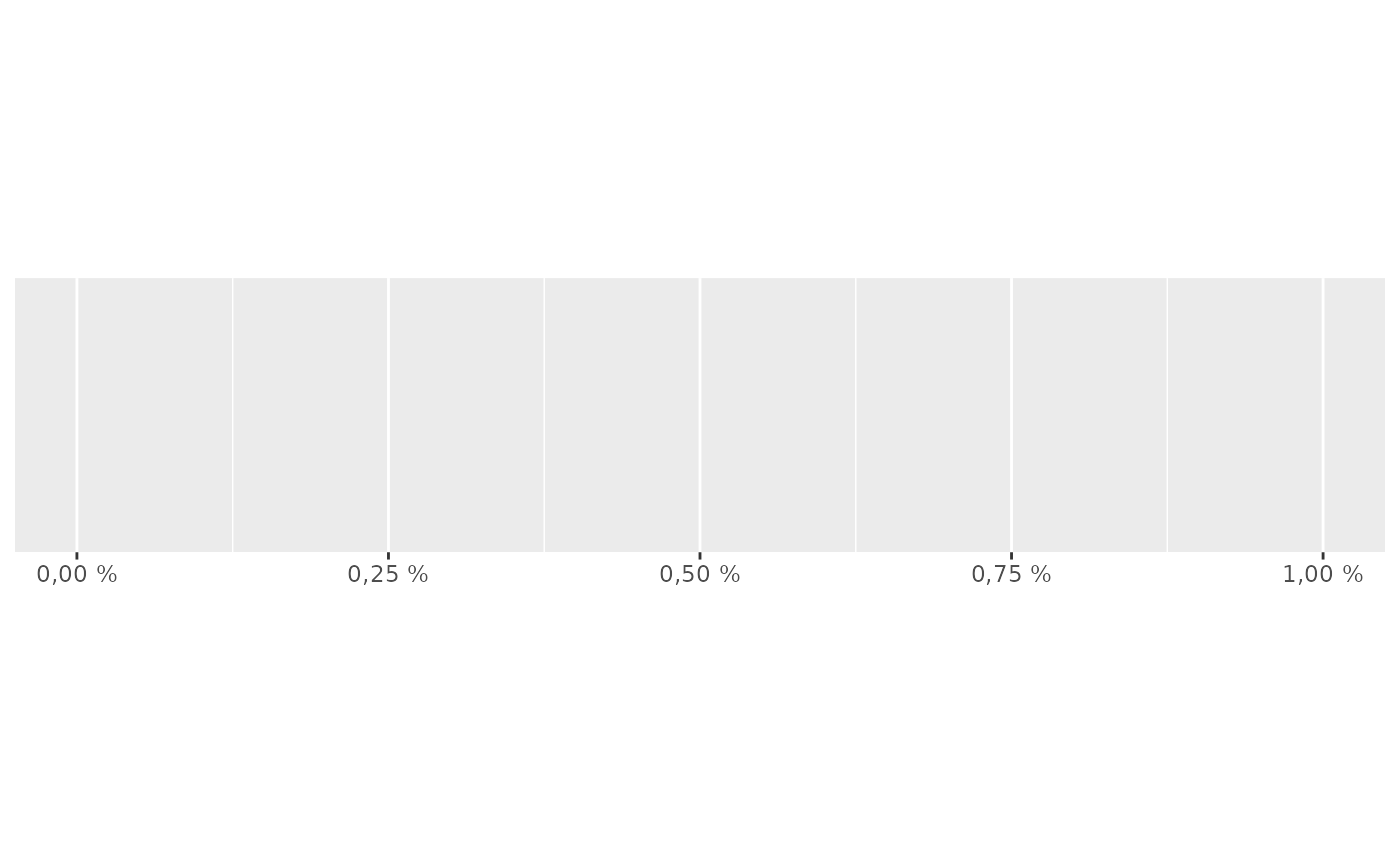Label percentages (2.5%, 50%, etc)

## Usage

label_percent(
accuracy = NULL,
scale = 100,
prefix = "",
suffix = "%",
big.mark = " ",
decimal.mark = ".",
trim = TRUE,
...
)

## Arguments

accuracy

A number to round to. Use (e.g.) 0.01 to show 2 decimal places of precision. If NULL, the default, uses a heuristic that should ensure breaks have the minimum number of digits needed to show the difference between adjacent values.

Applied to rescaled data.

scale

A scaling factor: x will be multiplied by scale before formatting. This is useful if the underlying data is very small or very large.

prefix

Additional text to display before the number. The suffix is applied to absolute value before style_positive and style_negative are processed so that prefix = "$" will yield (e.g.) -$1 and (\$1).

suffix

Additional text to display after the number.

big.mark

Character used between every 3 digits to separate thousands.

decimal.mark

The character to be used to indicate the numeric decimal point.

trim

Logical, if FALSE, values are right-justified to a common width (see base::format()).

...

Other arguments passed on to base::format().

## Value

All label_() functions return a "labelling" function, i.e. a function that takes a vector x and returns a character vector of length(x) giving a label for each input value.

Labelling functions are designed to be used with the labels argument of ggplot2 scales. The examples demonstrate their use with x scales, but they work similarly for all scales, including those that generate legends rather than axes.

Other labels for continuous scales: label_bytes(), label_dollar(), label_number_auto(), label_number_si(), label_ordinal(), label_parse(), label_pvalue(), label_scientific()

## Examples

demo_continuous(c(0, 1))
#> scale_x_continuous()demo_continuous(c(0, 1), labels = label_percent())
#> scale_x_continuous(labels = label_percent())# Use prefix and suffix to create your own variants
french_percent <- label_percent(
decimal.mark = ",",
suffix = " %"
)
demo_continuous(c(0, .01), labels = french_percent)
#> scale_x_continuous(labels = french_percent)